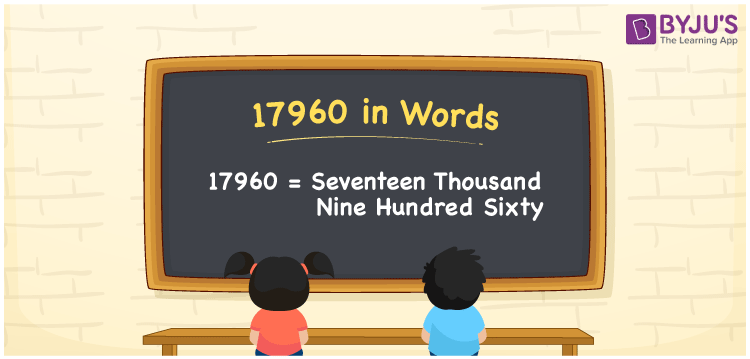# 17960 in Words

17960 in words is Seventeen Thousand Nine Hundred Sixty. For example, if you spend Rs. 17960 in a month, you can write, “I have spent Rs. Seventeen Thousand Nine Hundred Sixty in a month”. In general, the number name of any number can be written using the ones, tens, hundreds, and thousands place of a number. Thus, the place value chart is useful for writing the number 17960 in words.

 17960 in words Seventeen Thousand Nine Hundred Sixty Seventeen Thousand Nine Hundred Sixty in Numbers 17960

## 17960 in English Words## How to Write 17960 in Words?

We can convert 17960 to words using a place value chart. This can be done as follows. The number 17960 has 5 digits, so let’s make a chart that shows the place value up to 5 digits.

 Ten thousands Thousands Hundreds Tens Ones 1 7 9 6 0

Thus, we can write the expanded form as:

1 × Ten thousand + 7 × Thousand + 8 × Hundred + 6 × Ten + 0 × One

= 1 × 10000 + 7 × 1000 + 9 × 100 + 6 × 10 + 0 × 1

= 17960

= Seventeen Thousand Nine Hundred Sixty

17960 is the natural number that is succeeded by 17959 and preceded by 17961.

17960 in words – Seventeen Thousand Nine Hundred Sixty

Is 17960 an odd number? – No.

Is 17960 an even number? – Yes

Is 17960 a perfect square number? – No

Is 17960 a perfect cube number? – No

Is 17960 a prime number? – No

Is 17960 a composite number? – Yes

## Frequently Asked Questions on 17960 in Words

Q1

### How to write 17960 in words?

17960 in English is written as “Seventeen Thousand Nine Hundred Sixty”.
Q2

### Is the number 17960 an odd number?

No, the number 17960 is not an odd number.
Q3

### Is 17960 a composite number?

Yes, 17960 is a composite number.